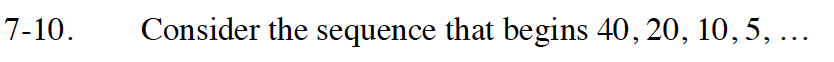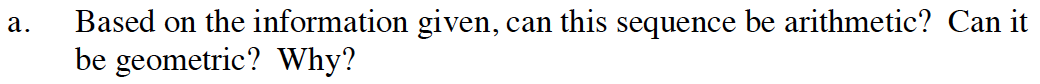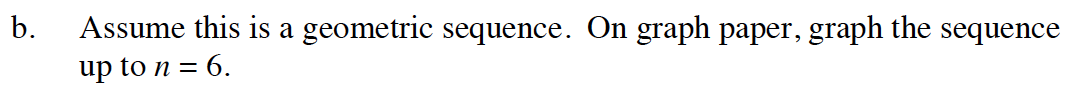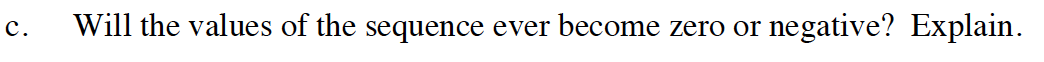Home > CC4 > Chapter 7 > Lesson 7.1.1 > Problem7-10

7-10.
1. Consider the sequence that begins 40, 20, 10, 5, … Homework Help ✎

1. Based on the information given, can this sequence be arithmetic? Can it be geometric? Why?

2. Assume this is a geometric sequence. On graph paper, graph the sequence up to n = 6.

3. Will the values of the sequence ever become zero or negative? Explain.Read the Math Notes box in Lesson 5.3.2.Start by making a table of values.No. Multiplying by 0.5 will never yield 0 unless the other factor is 0, which is impossible in this sequence.
When multiplying two positive numbers, a negative number can never be obtained.